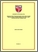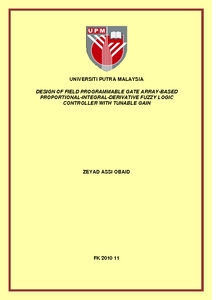# Design of field programmable gate array-based proportional-integral-derivative fuzzy logic controller with tunable ganin

## Citation

Obaid, Zeyad Assi (2010) Design of field programmable gate array-based proportional-integral-derivative fuzzy logic controller with tunable ganin. Masters thesis, Universiti Putra Malaysia.

## Abstract

Many of fuzzy control applications require real-time operation; higher density programmable logic devices such as Field Programmable Gate Array (FPGA) can be used to integrate large amounts of logic in a single IC. This thesis presents a design of improved Proportional-Integral-Derivative Fuzzy Logic Controller (PIDFC) with tunable gains method using FPGA. The PIDFC is designed as a PDFC and PIFC connected in parallel through a summer. To simplify the controller design, the PIFC is designed by accumulating the output of the PDFC. The benefits of doing so are twofold, as the number of rules that have to be written is reduced from 512 rules to 64 rules, and depending on two external signals, the controller is able to work as a PDFC, PIFC or PIDFC. The tuning gain block is designed at each input/output stage. This block involves a tuning via scaling the universe of discourse and is able to accept optimal scaling gains. The particle swarm optimization method (PSO) is used to obtain the optimal values of these gains. PIDFC is designed using VHDL language for implementation on FPGA device, and to employ the improved fuzzy algorithm that offer higher processing speed versus low utilization of chip resource. Two versions of the PIDFC are designed; the first one is 8-bits FPGA-based controller (8FBC), while the second one is 6-bits (6FBC) version for each inputs/output variables. To test the design, five case studies are used to test the controller in simulation environments in ModelSim and Matlab. The same design is coded in Matlab environment (MSBC) to enable a comparison with the FPGA-based design (FBC). PIDFC needs 16 clock cycles to complete one action. The simulation results showed that the 8FBC is superior to the 6FBC and its responses are much closer to or better to the MSBC or the results in the literature. 8FBC is able to produce an action in 0.3 μs after input latching with maximum frequency of 40 MHz. Therefore, the PIDFC will be able to control a wide range of the systems with high sampling rate.Preview
PDF
FK 2010 11R.pdfView Item